Updating search results...

# 422 Results

View
Selected filters:
• math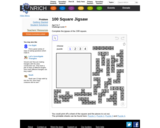Unrestricted Use
CC BY
Rating
0.0 stars

ThisNrich jigsaw a great way to reinforce children's understanding of the sequences contained within the hundred square even if they have seen it before. It could be used as an exploratory tool for children who haven't met the 0 - 99 hundred square before (puzzles 2 and 4 in the interactivity), or it could play a part in assessing their understanding of it, if they have already met it.

Subject:
Mathematics
Numbers and Operations
Material Type:
Activity/Lab
Interactive
06/09/2021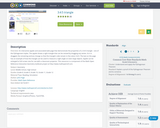Rating
0.0 stars

An interactive applet and associated web page that demonstrate the properties of a 3:4:5 triangle - one of the Pythagorean triples. The applet shows a right triangle that can be resized by dragging any vertex. As it is dragged, the remaining vertices change so that the triangle's side remain in the ration 3:4:5. The text on the page has an example of how the triangle can be used to measure a right angle on even large objects. Applet can be enlarged to full screen size for use with a classroom projector. This resource is a component of the Math Open Reference Interactive Geometry textbook project at http://www.mathopenref.com.

Subject:
Mathematics
Material Type:
Activity/Lab
Interactive
Author:
John Page
08/04/2020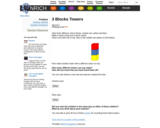Unrestricted Use
CC BY
Rating
0.0 stars

Students explore combinations using colored blocks in this Nrich activity. The activity emphasizes mathematical reasoning and discourse.

Subject:
Mathematics
Material Type:
Activity/Lab
05/31/2021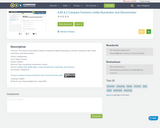Conditional Remix & Share Permitted
CC BY-NC-SA
Rating
0.0 stars

This resource will enable students to determine flawed reasoning in a fraction comparison with unlike numerators and denominators.

Subject:
Mathematics
Material Type:
Activity/Lab
Assessment
Lesson
Author:
Amanda Ray
KIm Quintyne
Linda Schoenbrodt
08/04/2020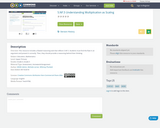Conditional Remix & Share Permitted
CC BY-NC-SA
Rating
0.0 stars

This resource includes a flawed reasoning task that reflects 5.NF.5. Students must find the flaw in an argument and present it correctly. Then, they should provide a reasoning behind their thinking.

Subject:
Mathematics
Material Type:
Assessment
Homework/Assignment
Author:
Michele Lerner
Whitney Plunkett
08/04/2020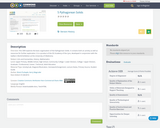Conditional Remix & Share Permitted
CC BY-NC-SA
Rating
0.0 stars

This OER explores the basic organization of the Pythagorean Solids. It contains both an activity as well as resources for further exploration. It is a product of the OU Academy of the Lynx, developed in conjunction with the Galileo's World Exhibition at the University of Oklahoma.

Subject:
Mathematics
Material Type:
Activity/Lab
Homework/Assignment
Lecture Notes
Author:
Brent Purkaple
Kerry Magruder
08/06/2020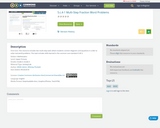Conditional Remix & Share Permitted
CC BY-NC-SA
Rating
0.0 stars

This resource includes two multi-step tasks where students connect diagrams and equations in order to solve real world problems. This task includes skills learned in the common core standard 5.NF.2

Subject:
Mathematics
Numbers and Operations
Material Type:
Activity/Lab
Author:
Whitney Plunkett
08/03/2020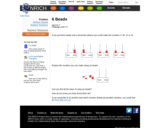Unrestricted Use
CC BY
Rating
0.0 stars

This Nrich problem is a good, yet simple, activity that can get pupils thinking hard about numerals, numbers and place value. It also provides a context for discussing different ways of recording and for encouraging systematic working.

Subject:
Mathematics
Material Type:
Activity/Lab
06/11/2021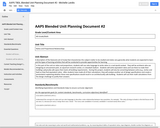Conditional Remix & Share Permitted
CC BY-NC-SA
Rating
0.0 stars

In this part of the unit on ratios and proportions, students will use ratio language to write ratios in a real-world context.  They will be architects who are designing an amusement park, an aquarium research center, or a baseball stadium.  Students will write equivalent ratios and use them to make their building a physically safe space that meets all building requirements.  During this project, students will also be converting between units (e.g., inches and feet) as they find equivalent ratios.  After students have made all of their calculations, they will analyze another set of design specifications and create a presentation explaining whether these new specifications would result in an architecturally safe building.  Students will use their math calculations from the design challenge to justify their answers.

Subject:
Mathematics
Material Type:
Unit of Study
Provider:
Michigan Virtual
Author:
Michelle Landis
03/24/2017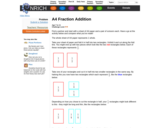Unrestricted Use
CC BY
Rating
0.0 stars

This Nrich activity enables pupils to help to develop their concept of fractions and begin to add fractions with the same denominator and denominators that are multiples of the same number. It provides a context within which pupils can explore and reason about properties of fractions.

Subject:
Mathematics
Material Type:
Activity/Lab
06/17/2021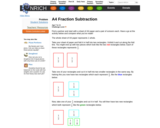Unrestricted Use
CC BY
Rating
0.0 stars

This Nrich activity enables pupils to help to develop their concept of fractions and begin to subtract fractions with the same denominator and denominators that are multiples of the same number. It provides a context within which pupils can explore and reason about properties of fractions.

Subject:
Mathematics
Material Type:
Activity/Lab
06/17/2021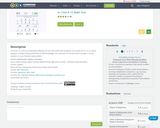Conditional Remix & Share Permitted
CC BY-NC-SA
Rating
0.0 stars

A+ Click is an interactive collection of more than 3700 math problems and answers for K-1 K-12 school program. It defines the personal level of math knowledge. You move up into the next level if you give 5 correct answers in a row. Practice makes perfect.

Subject:
Algebra
Geometry
Mathematics
Numbers and Operations
Material Type:
Activity/Lab
Assessment
Game
Lecture Notes
Author:
Igor Kokcharov
08/04/2020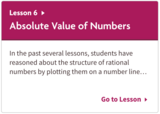Unrestricted Use
CC BY
Rating
0.0 stars

Unit 7: Rational Numbers
Lesson 6: Absolute Value of Numbers

In the past several lessons, students have reasoned about the structure of rational numbers by plotting them on a number line and noting their relative positions and distances from zero. They learned that opposite numbers have the same distance from zero. Students now formalize the concept of a number’s magnitude with the term absolute value. They learn that the absolute value of a number is its distance from zero, which means that opposite numbers have the same absolute value. Students reason abstractly about the familiar contexts of temperature and elevation using the concept and notation of absolute value (MP2).

Subject:
Mathematics
Material Type:
Activity/Lab
05/18/2021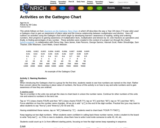Unrestricted Use
CC BY
Rating
0.0 stars

In this Nrich article, Alf outlines six activities using the Gattegno chart which begin by developing learners' awareness of reading, writing and ordering numbers, then progress to gaining awareness of multiplication facts, multiplication and division by 10, onto fractions as operations and, finally, to finding percentages of any number.

Subject:
Mathematics
Material Type:
Activity/Lab
Author:
Article Alf Coles
06/18/2021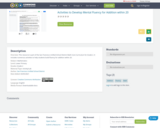Unrestricted Use
CC BY
Rating
0.0 stars

This resource is part of the San Francisco Unified School District Math Core Curriculum for Grade 2. It includes numerous activities to help students build fluency for addition within 20.

Subject:
Mathematics
Material Type:
Activity/Lab
05/13/2021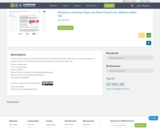Unrestricted Use
CC BY
Rating
0.0 stars

This resource is part of the San Francisco Unified School District Math Core Curriculum for Grade 2. It includes numerous activities to help students build fluency for addition within 100.

Subject:
Mathematics
Material Type:
Activity/Lab
Teaching/Learning Strategy
05/13/2021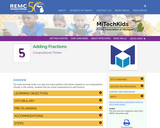Conditional Remix & Share Permitted
CC BY-NC-SA
Rating
0.0 stars

The math learning center is an app and online platform that allows students to use manipulatives virtually. In this activity, students will use virtual manipulatives to add fractions.

Subject:
Education
Educational Technology
Material Type:
Activity/Lab
Game
Interactive
Module
Provider:
REMC Association of Michigan
Provider Set:
MiTechKids
Author:
REMC Association of Michigan
09/25/2023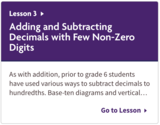Unrestricted Use
CC BY
Rating
0.0 stars

Unit 5: Arithmetic in Base Ten
Lesson 3: Adding and Subtracting Decimals with Few Non-Zero Digits

As with addition, prior to grade 6 students have used various ways to subtract decimals to hundredths. Base-ten diagrams and vertical calculations are likewise used for subtracting decimals. “Unbundling,” which students have previously used to subtract whole numbers, is a key idea here. They recall that a base-ten unit can be expressed as another unit that is 1/10 its size. For example, 1 tenth can be “unbundled” into 10 hundredths or into 100 thousandths. Students use this idea to subtract a larger digit from a smaller digit when both digits are in the same base-ten place, e.g., 0.012 - 0.007. Rather than thinking of subtracting 7 thousandths from 1 hundredth and 2 thousandths, we can view the 1 hundredth as 10 thousandths and subtract 7 thousandths from 12 thousandths.

Unbundling also suggests that we can write a decimal in several equivalent ways. Because 0.4 can be viewed as 4 tenths, 40 hundredths, 400 thousandths, or 4,000 ten-thousandths, it can also be written as 0.40, 0.400, 0.4000, and so on; the additional zeros at the end of the decimal do not change its value. They use this idea to subtract a number with more decimal places from one with fewer decimal places (e.g., 2.5 - 1.028). These calculations depend on making use of the structure of base-ten numbers (MP7).

The second activity is optional; it gives students additional opportunities to practice summing decimals.

Subject:
Mathematics
Material Type:
Activity/Lab
04/14/2021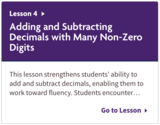Unrestricted Use
CC BY
Rating
0.0 stars

Unit 5: Arithmetic in Base Ten
Lesson 4: Adding and Subtracting Decimals with Many Non-Zero Digits

This lesson strengthens students’ ability to add and subtract decimals, enabling them to work toward fluency. Students encounter longer decimals (beyond thousandths), find missing addends, and work with decimals in the context of situations. They decide which operation (addition or subtraction) to perform and which strategy to use when finding sums and differences. They also reinforce the idea that we can express a decimal in different but equivalent ways, and that writing additional zeros after the last non-zero digit in a decimal does not change its value. Students use this understanding to practice subtracting numbers with more decimal places from those with fewer decimal places (e.g., 1.9 - 0.4563).

To solve these problems, students must lean heavily on their understanding of base-ten numbers (MP7). Given problems such as 7 - ? = 3.4567 and 0.404 + ? = 1, they need to think carefully about the meaning of each place value, the meaning of addition and subtraction, and potential paths toward the solution. Along the way, they also begin to see patterns in the calculations, which enables them to become increasingly fluent in finding sums and differences (MP8).

Subject:
Mathematics
Material Type:
Activity/Lab
04/14/2021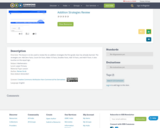Only Sharing Permitted
CC BY-NC-ND
Rating
0.0 stars

The lesson is to be used to review the six addition strategies the first grade class has already learned. The strategies are: Add Zero Facts, Count On Facts, Make 10 Facts, Doubles Facts, Add 10 Facts, and Add 9 Facts. It also touches on the equal sign.

Subject:
Mathematics
Material Type:
Lesson
Student Guide